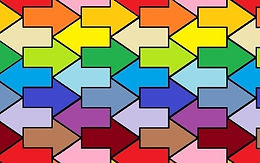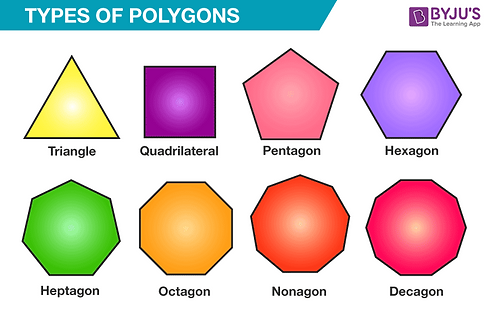top of page

# Tessellation

Lesson Overview

In this lesson, students will learn how to use programming to create a tessellation made by a regular hexagon automatically. To finish the task, they need to acquire the concept of tessellation, apply the properties of regular polygons, and comprehend the relationship between interior and exterior angles. The problem-solving process will motivate students to master how to calculate the degrees of the interior and exterior angles of a regular polygon and figure out what is a good way to draw different regular hexagons to form a tessellation, as well as other fundamental CT concepts and practices (e.g., loops, subroutines, decomposition).

### Prior Knowledge

• Mathematics: definition of a regular polygon; coordinate system

• Scratch: know how to move and turn the direction of the sprite, able to use pen function to draw

### Learning Objectives

• To acquire the mathematics concept of tessellation and the properties of regular polygons.

• To know how to create tessellation by programming:

• To use the loop and conditional blocks to draw a regular hexagon;

• To employ a random block to jump out of the loop of drawing a single regular hexagon;

• To experience the meaning of automation and practice of decomposition.

### Mathematics & CT Outcomes

1. Mathematics: properties of polygons, the relationship between interior angles and exterior angles in a polygon, the concept of tessellation

2. CT Skills: loops (repeat XX; forever); conditionals (if XX then XX), subroutines (create my blocks); sequence; automation (draw tessellation shapes automatically); decomposition (break down the tessellation process into three small problems)

### Teaching Resources

The Desmos Activity used in this Task.

## Lesson Details

What is Tessellation?

Tessellation is a plane using one or more geometric shapes, with NO overlaps and NO gaps. Usually, each tessellation will have a repeating pattern.### Make a guess!

For a regular polygon, which one could form a tessellation?Could you draw the figure if it can form a tessellation? Try to draw it on paper with your pen!

Actually, we can write programs to help us create a tessellation easier! Watch the video to see how scratch does the drawing process!

Based on the requirements shown in the video, try to choose some code to create a special Tessellation!

Click Remix: https://scratch.mit.edu/projects/556178337/

### Guiding Questions

1. What can you observe in the video? Are there any relations to the codes of previous activity?

2. Are there any patterns to the arrangements of these hexagons?

3. How does the program do this? What should it happen if the arrow touches the edge?

4.  Could you change the program to show a pentagon can/cannot tesselate?

5.  Is it possible to draw a tessellation without drawing overlapping figures (using this method)?

6.  Could you try to create a non-regular figure that has tessellation?

A sample code. Click the image to view the program

#### Students' WorkIn this work, there are three key points:

1. Drawing a regular hexagon: setting equal side lengths and rotating the degree of an exterior angle clockwise each time;

2. Detecting whether the pen touches the edge: if it touches the edge, the pen returns to the starting point;

3. Using a random number greater than 5 to determine the number of sides to draw:  makes it possible to complete the drawing of a regular hexagon and to jump out of the loop of drawing a single regular hexagon and redraw the hexagon in a different direction.

#### Possible Challenges and Misconceptions

##### A misunderstanding of the properties of geometric tessellation leads to the misconception that all polygons can be tessellated.In this work, the student considered the three key problems that needed to be solved in this lesson (i.e., drawing a regular polygon, checking whether the edge is touched and setting a random number to jump out of the loop for drawing a single regular polygon). What’s more, the student was able to generalise the solution so that the program could automatically draw polygons with different numbers of sides.

However, where this work could be improved is that before running the tessellation program, the variable [number of sides] should be checked to see if the tessellation conditions are met (e.g., number of sides 3, 4, 6; see the figure), otherwise the shapes will overlap (e.g., number of sides 5, 7, 8; see the figure) and the tessellation will not be completed.## Acknowledgement

The author would like to thank Hin Cheung LAW for designing this lesson and appreciate all the anonymous teachers and students who participated in this research.

bottom of page